# How to Calculate and Solve for Linear Density | Crystal Structures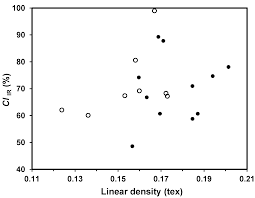The image above represents linear density.

To compute for linear density, two essential parameters are needed and these parameters are Number of Atoms Centered on Direction Vector (N) and Length of Direction Vector (L).

The formula for calculating linear density:

LD = N/L

Where:

LD = Linear Density
N = Number of Atoms Centered on Direction Vector
L = Length of Direction Vector

Given an example;
Find the linear density when the number of atoms centered on direction vector is 10 and the length of direction vector is 2.

This implies that;

N = Number of Atoms Centered on Direction Vector = 10
L = Length of Direction Vector = 2

LD = N/L
LD = 10/2
LD = 5

Therefore, the linear density is 5 atoms/m.

Calculating the Number of Atoms Centered on Direction Vector when the Linear Density and the Length of Direction Vector is Given.

N = LD x L

Where:

N = Number of Atoms Centered on Direction Vector
LD = Linear Density
L = Length of Direction Vector

Let’s solve an example;
Find the number of atoms centered on direction vector when the linear density is 6 and the length of direction vector is 5.

This implies that;

LD = Linear Density = 6
L = Length of Direction Vector = 5

N = LD x L
N = 6 x 5
N = 30

Therefore, the number of atoms centered on direction vector is 30 atoms.

Calculating the Length of Direction Vector when the Linear Density and the Number of Atoms Centered on Direction Vector is Given.

L = N / LD

Where:

L = Length of Direction Vector
LD = Linear Density
N = Number of Atoms Centered on Direction Vector

Let’s solve an example;
Find the length of direction vector when the linear density is 12 and the number of atoms centered on direction vector is 72.

This implies that;

LD = Linear Density = 12
N = Number of Atoms Centered on Direction Vector = 72

L = N / LD
L = 72 / 12
L = 6

Therefore, the length of direction vector is 6 m.

Nickzom Calculator – The Calculator Encyclopedia is capable of calculating the linear density.

To get the answer and workings of the linear density using the Nickzom Calculator – The Calculator Encyclopedia. First, you need to obtain the app.

You can get this app via any of these means:

You can also try the demo version via https://www.nickzom.org/calculator

Apple (Paid) – https://itunes.apple.com/us/app/nickzom-calculator/id1331162702?mt=8
Once, you have obtained the calculator encyclopedia app, proceed to the Calculator Map, then click on Materials and Metallurgical under Engineering.Now, Click on Crystal Structures under Materials and Metallurgical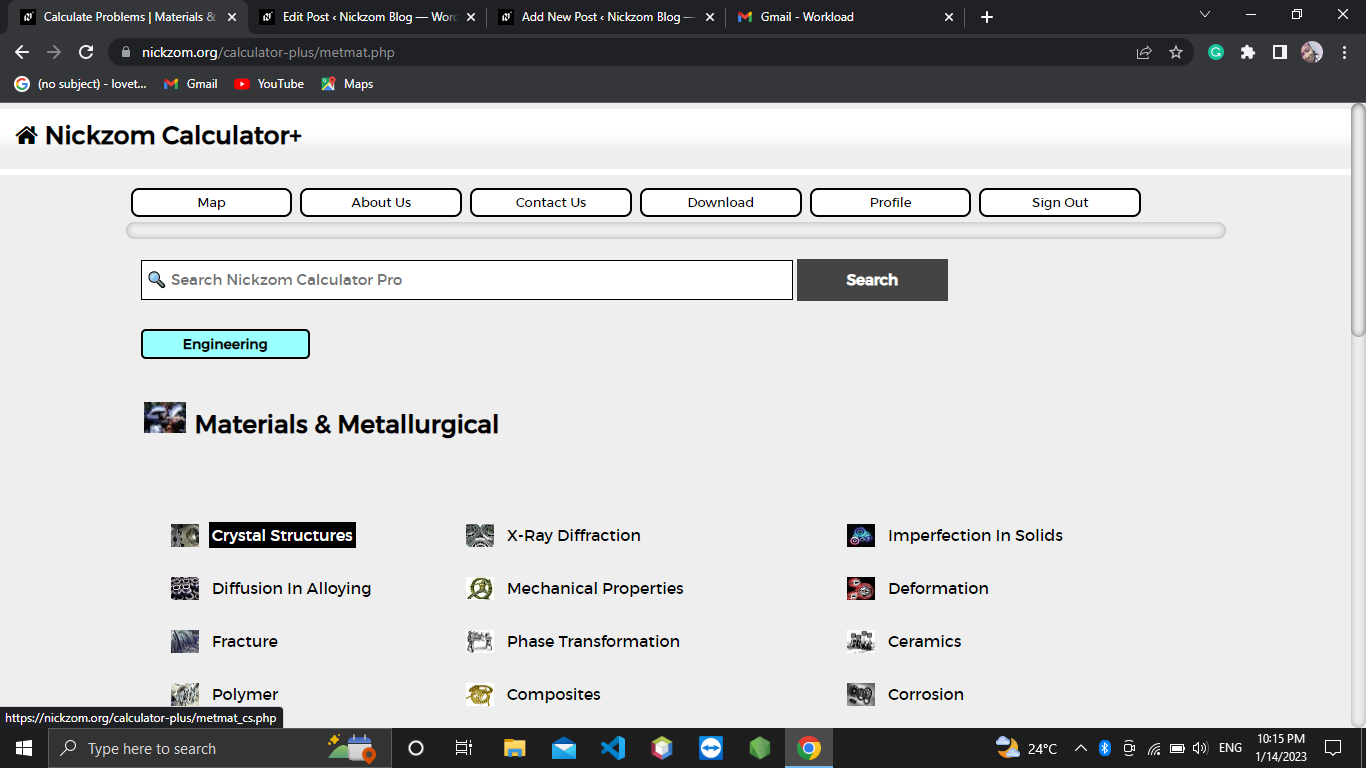Now, Click on Linear Density under Crystal Structures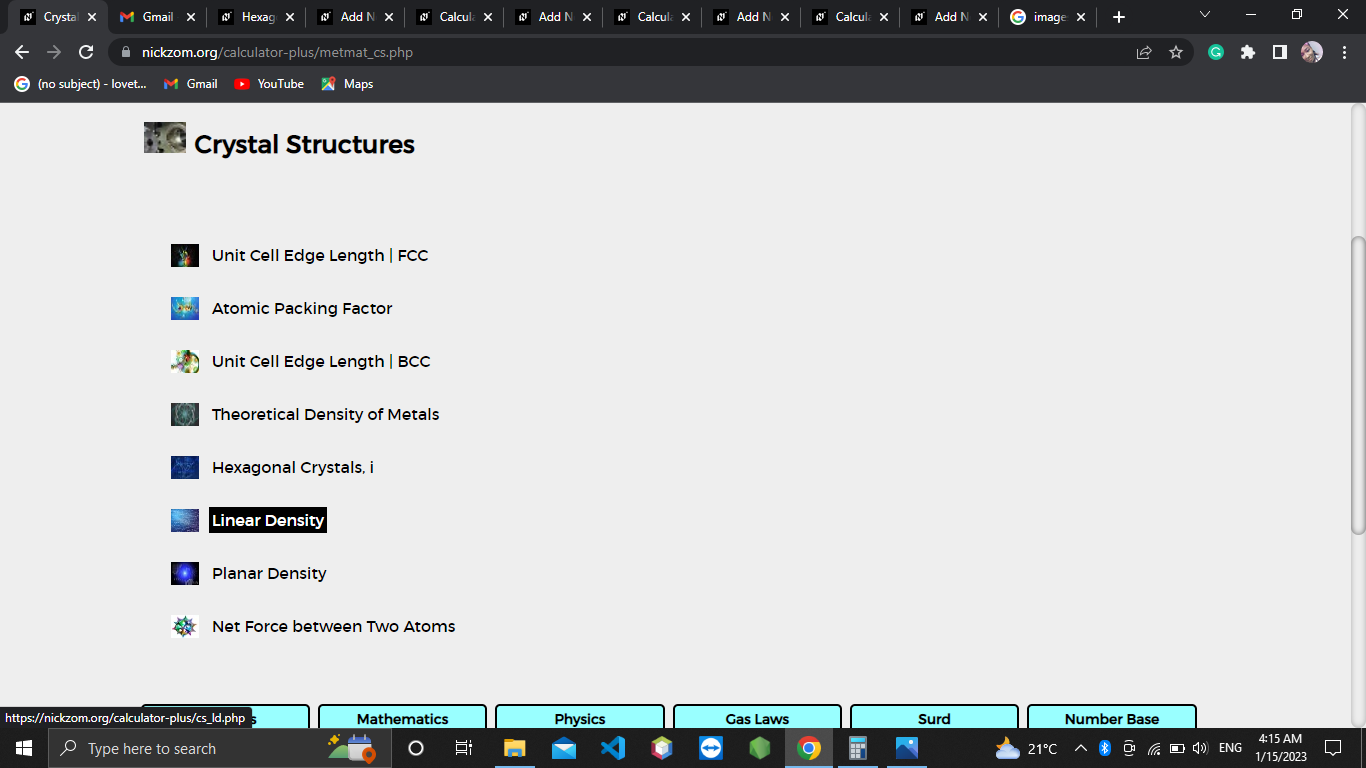The screenshot below displays the page or activity to enter your value, to get the answer for the linear density according to the respective parameter which is the Number of Atoms Centered on Direction Vector (N) and Length of Direction Vector (L).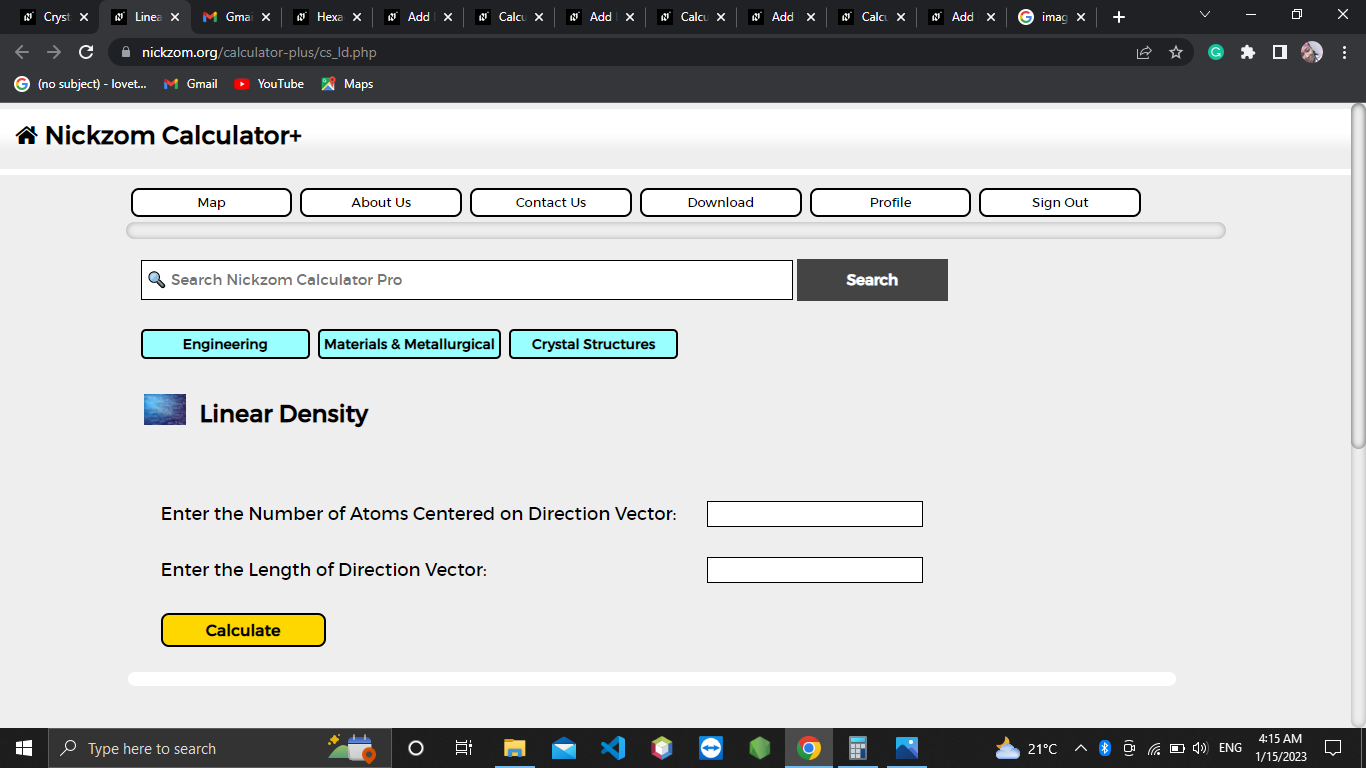Now, enter the values appropriately and accordingly for the parameter as required by the Number of Atoms Centered on Direction Vector (N) is 10 and Length of Direction Vector (L) is 2.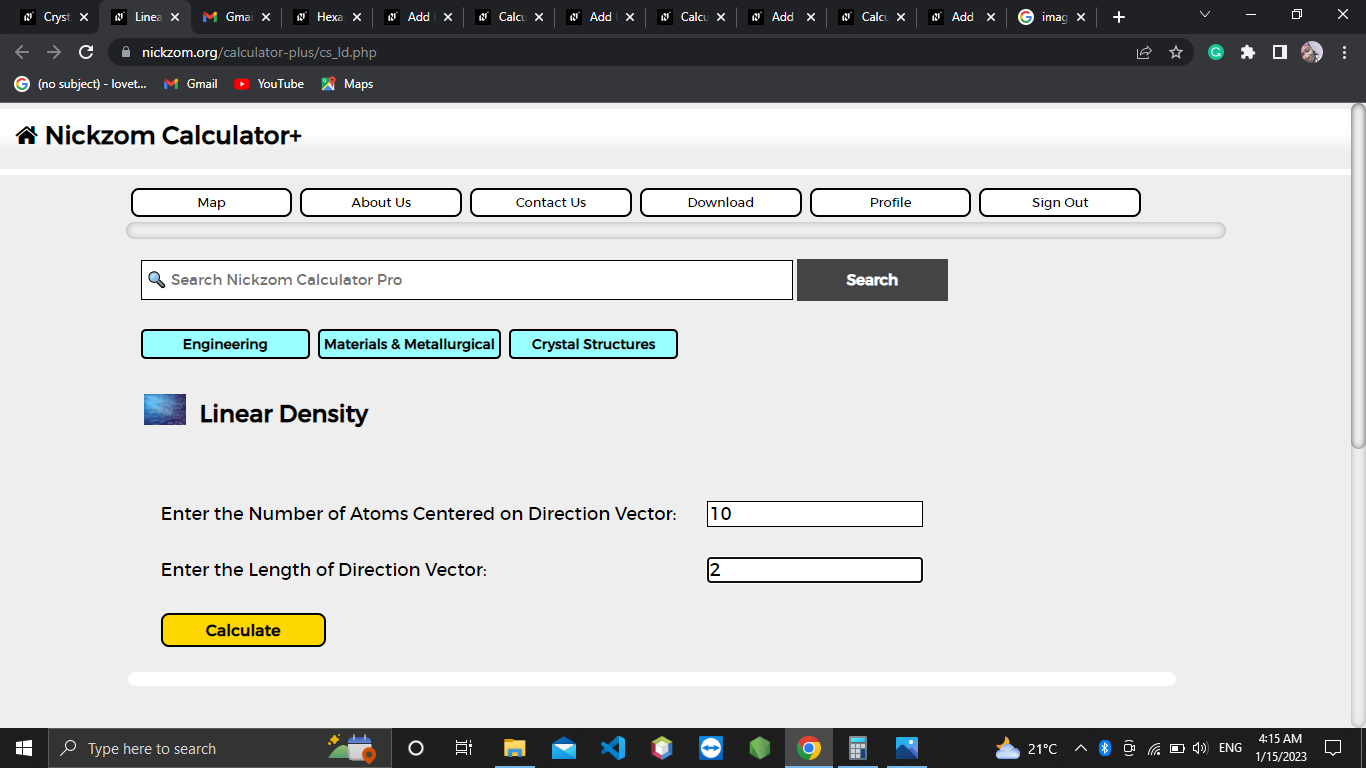Finally, Click on Calculate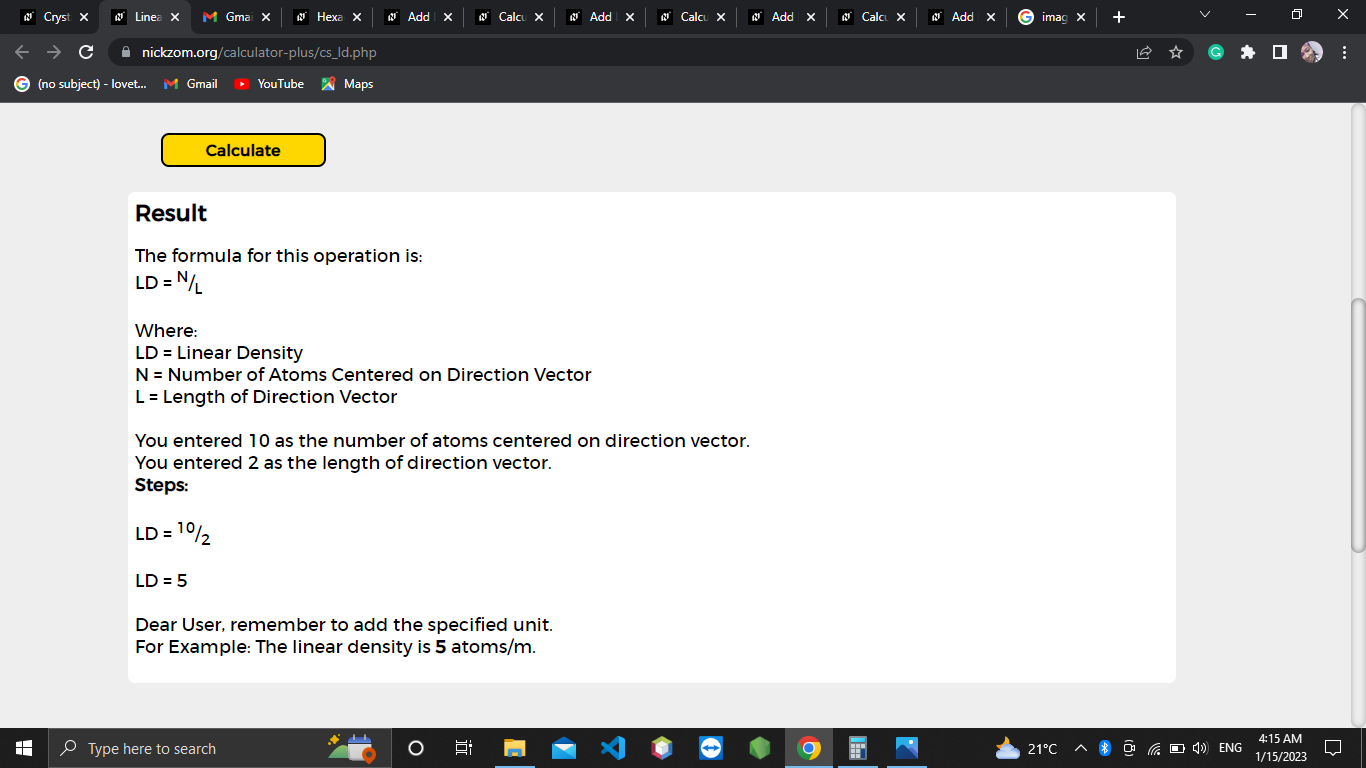As you can see from the screenshot above, Nickzom Calculator– The Calculator Encyclopedia solves for the linear density and presents the formula, workings and steps too.